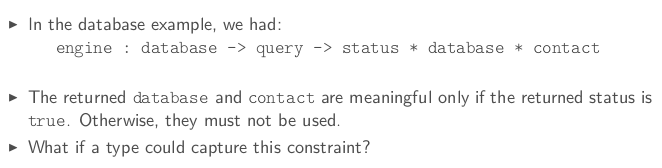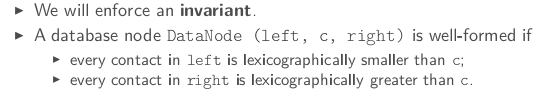# [OCaml MOOC] week3: MORE ADVANCED DATA STRUCTURES

[TOC]

Last week, we only defined flat data structures which are nice to aggregate values but quite limited when you try to structure values.

This week: algebraic datatypes.

# 1. TAGGED VALUES⇒ change the return type to a type `query_result`, which can be either of these:

• an error
• a new database (in case of successful insertion/deletion)
• a contact and its index (in case of successful search)

in ocaml, can define such a type (called sum type) by :

```# type query_result =
| Error
| NewDatabase of database
| FoundContact of contact*int;;
```

More generally, to define disjoint union of types:

```type some_type_identifier =
| SomeTag of some_type
| ...
| SomeTag of some_type
```

tag must start with uppercase letter

Taga are also called conscturcors, grammar is like java constructors: `SomeTag (some_expr, ..., some_expr)` (the parenthesis can be omitted if only 1 expr is required)

enumeration:

`type color = Black | Gray | White;;`

### observing tagged values

must prvide an expression for each possible case of the value. A case is described by a pattern:
`SomeTag (some_pattern, ..., some_pattern)`

A branch is composed of a pattern an an expr separated by an arrow. `some_pattern -> some_expr`

pattern matching is a seq of branches:

```match some_expr with
| some_pattern -> some_expr
|...
| some_pattern -> some_expr
```

example:

```let engine db query =
match query with
| Insert contact -> insert db contact
| Delete contact -> delete db contact
| Search name -> search db name;;
```

synatactic shortcut: function keyword (for functions with only 1 argument)pitfalls

• ill-typed pattern
• non-exhaustive case analysis

These errors can be caught by the checker.

# 2. RECURSIVE TYPES

data structures with unbounded depth, ie, list/tree.

For example, an integer list can be defined as:

```# type int_list =
| EmptyList
| SomeElement of int * int_list;;
type int_list = EmptyList | SomeElement of int * int_list
```

in the machine:functions on such datastruct usually use pattern matching:

```# let rec length = function
|EmptyList -> 0
|SomeElement (x,l) -> 1 + length l;;
val length : int_list -> int = <fun>
```

The predefined type in ocaml: `t list`

• empty list: `[]` ( `[]` is just a special tage corresponding to EmptyList)
• head and tail: `i::r` ( `::` is just a special tage corresponding to SomeElement)
• a list can be defined by enumeration: `[some_expr; ...; some_expr]`
• list concatenation: `@`
```# let rec length = function
| [] -> 0
| x::xs -> 1 + length xs;;
val length : 'a list -> int = <fun>
# length [1;2;3;];;
- : int = 3
# let rec rev = function
| [] -> []
| x::xs -> (rev xs)@[x];;
val rev : 'a list -> 'a list = <fun>
# rev [1;2;3;4];;
- : int list = [4; 3; 2; 1]
```

the `rev` function above has quad-complexity → here is the tail rec version:

```# let rec rev_aux accu = function
| [] -> accu
| x::xs -> rev_aux (x::accu) xs;;
val rev_aux : 'a list -> 'a list -> 'a list = <fun>
# let rev l = rev_aux [] l;;
val rev : 'a list -> 'a list = <fun>
```

# 3. TREE-LIKE VALUES

the database type is formed in a (binary)tree-like fashion:

```# type database =
| NoContact
| DataNode of database * contact * database;;
type database = NoContact | DataNode of database * contact * database
```

impose the BST invariant:Now the functions insert/search/delete is BST fashion:

# 4. CASE STUDY: A STORY TELLER

type-directed programming: writing the right type declaration is half success.

define a story type (and other types:

```type story = {
context: context;
perturbation: event;
adventure: event list;
conclusion: context;
}

and context = {characters: character list}

and character = {
name: string;
state: state;
location: location;
}

and event =
| Change of character * state
| Action of character * action

and state = Happy | Hungry

and action = Eat | GoToRestaurant

and location = Appartment | Restaurant;;
```

# 5. POLYMORPHIC ALGEBRAIC DATATYPES

parametric programming: example — list is parametrized by the element type.
Hence in `List` module contains polymorphic functions.

Good for code reuse.

define your own polymorphic types, using '`a` to indicate unkonw types:

`type ('a1,...,1aN) some_type_identifier = some_type`

example:

```type 'a option =
| None
| Some of 'a;;
type ('a, 'b) either =
| Left of 'a
| Right of 'b;;
type square = {dimension: int);;
type circle = {radius: int);;
type shape = (square, circle) either;;
```

another example: bst:

```type 'a bst =
| Empty
| Node of 'a bast * a' * 'a bst ;;

let rec insert x = function
| Empty -> Node (Empty, x, Empty)
| Node (l, y, r) ->
if x=y then Node (l,y,r)
else if x<y then Node (insert x l, y, r)
else Node (l, y, insert x r);;
```

# 6. ADVANCED TOPICS

### precise typing

when 2 types have the same structure but different semantical meaning: a sum type with only one constructor can be useful to distinguish them.

example:

```type euro = Euro of float;;
type dollar = Dollar of float;;
let euro_of_dollar (Dollar d) = Euro (d /. 1.33);;
let x = Dollar 4;;
let y = Euro 5;;
let valid_comparison = (euro of dollar x < y)
```

### disjunctive patterns

Use or-patterns to factorize branches into a unique branch:

`some_pattern_1 | some_pattern_2` means observation of either pattern 1 or pattern 2.

constraint: both must contain the same identifiers.

ex:

```let remove_zero_or_one_head = function
| 0::xs | 1::xs -> xs
| l -> l
let remove_zero_or_one_head' = function

| (0|1)::xs -> xs
| l -> l
```

### as-patterns

convenient ot name a matched component: `some_pattern as x` ( if the value can be observed using some_pattern, name it x)

ex.

```let rec duplicate_head_at_the_end = function
| [] -> []
| (x::_) as l -> l @[x]
```

### guard: pattern matching branch using `when`

a guard (some bool-expression) can add an extra constraint to a pattern:

ex.

```let rec push_max_at_the_end = function
| ([] | [_]) as l -> l
| x::((y::_) as l) when x<=y -> x::(push_max_at_the_end l)
| x::y::ys -> y::push_max_at_the_end (x::ys);; (*when x>y, should permuate x and y*)
```

#### Part 4 of series «Introduction to Functional Programming in OCaml»：

comments powered by Disqus